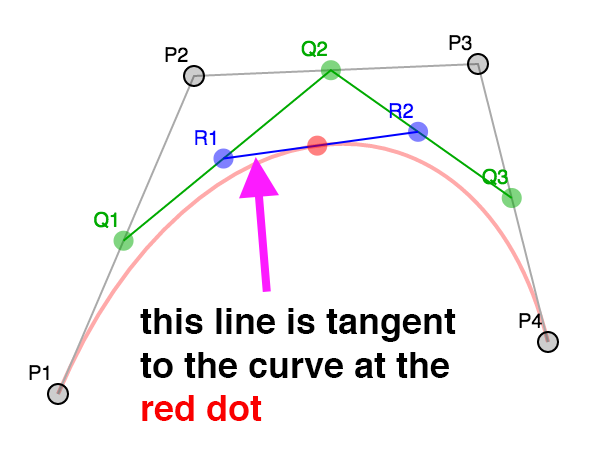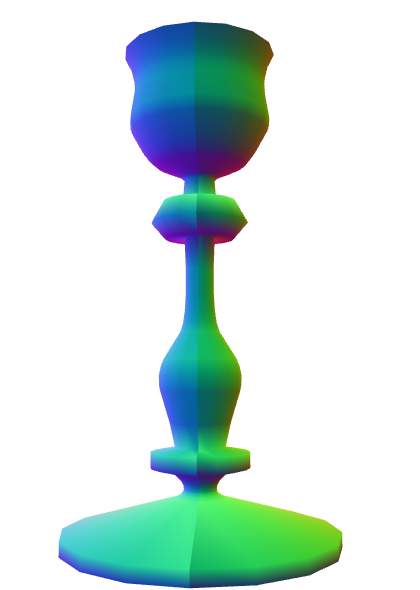# WebGLFundamentals.org

Fix, Fork, Contribute

# WebGL 三维几何加工

```invT = (1 - t)
P = P1 * invT^3 +
P2 * 3 * t * invT^2 +
P3 * 3 * invT * t^2 +
P4 * t^3
```

``````function getPointOnBezierCurve(points, offset, t) {
const invT = (1 - t);
return v2.add(v2.mult(points[offset + 0], invT * invT * invT),
v2.mult(points[offset + 1], 3 * t * invT * invT),
v2.mult(points[offset + 2], 3 * invT * t * t),
v2.mult(points[offset + 3], t * t  *t));
}
``````

``````function getPointsOnBezierCurve(points, offset, numPoints) {
const points = [];
for (let i = 0; i < numPoints; ++i) {
const t = i / (numPoints - 1);
points.push(getPointOnBezierCurve(points, offset, t));
}
return points;
}
``````

``````function flatness(points, offset) {
const p1 = points[offset + 0];
const p2 = points[offset + 1];
const p3 = points[offset + 2];
const p4 = points[offset + 3];

let ux = 3 * p2 - 2 * p1 - p4; ux *= ux;
let uy = 3 * p2 - 2 * p1 - p4; uy *= uy;
let vx = 3 * p3 - 2 * p4 - p1; vx *= vx;
let vy = 3 * p3 - 2 * p4 - p1; vy *= vy;

if(ux < vx) {
ux = vx;
}

if(uy < vy) {
uy = vy;
}

return ux + uy;
}
``````

``````function getPointsOnBezierCurveWithSplitting(points, offset, tolerance, newPoints) {
const outPoints = newPoints || [];
if (flatness(points, offset) < tolerance) {

// 将它加入点队列中
outPoints.push(points[offset + 0]);
outPoints.push(points[offset + 3]);

} else {

// 拆分
const t = .5;
const p1 = points[offset + 0];
const p2 = points[offset + 1];
const p3 = points[offset + 2];
const p4 = points[offset + 3];

const q1 = v2.lerp(p1, p2, t);
const q2 = v2.lerp(p2, p3, t);
const q3 = v2.lerp(p3, p4, t);

const r1 = v2.lerp(q1, q2, t);
const r2 = v2.lerp(q2, q3, t);

const red = v2.lerp(r1, r2, t);

// 求前半段的点
getPointsOnBezierCurveWithSplitting([p1, q1, r1, red], 0, tolerance, outPoints);
// 求后半段的点
getPointsOnBezierCurveWithSplitting([red, r2, q3, p4], 0, tolerance, outPoints);

}
return outPoints;
}
``````

``````function simplifyPoints(points, start, end, epsilon, newPoints) {
const outPoints = newPoints || [];

// 找到离最后两点距离最远的点
const s = points[start];
const e = points[end - 1];
let maxDistSq = 0;
let maxNdx = 1;
for (let i = start + 1; i < end - 1; ++i) {
const distSq = v2.distanceToSegmentSq(points[i], s, e);
if (distSq > maxDistSq) {
maxDistSq = distSq;
maxNdx = i;
}
}

// 如果距离太远
if (Math.sqrt(maxDistSq) > epsilon) {

// 拆分
simplifyPoints(points, start, maxNdx + 1, epsilon, outPoints);
simplifyPoints(points, maxNdx, end, epsilon, outPoints);

} else {

// 添加最后两个点
outPoints.push(s, e);
}

return outPoints;
}
``````

`v2.distanceToSegmentSq` 是计算点到线段距离平方的一个方法， 使用距离平方的原因是比使用实际距离要快一些，因为我们值管线最远距离所以和实际距离的效果相同。``````<path fill="none" stroke-width="5" d="
m44,434
c18,-33 19,-66 15,-111
c-4,-45 -37,-104 -39,-132
c-2,-28 11,-51 16,-81
c5,-30 3,-63 -36,-63
"/>
``````

``````        ___
44, 371,   |
62, 338,   | 第一个曲线
63, 305,___|__
59, 260,___|  |
55, 215,      | 第二个曲线
22, 156,______|__
20, 128,______|  |
18, 100,         | 第三个曲线
31,  77,_________|__
36,  47,_________|  |
41,  17,            | 第四个曲线
39, -16,            |
0, -16,____________|
``````

``````// 获取所有片段的点
function getPointsOnBezierCurves(points, tolerance) {
const newPoints = [];
const numSegments = (points.length - 1) / 3;
for (let i = 0; i < numSegments; ++i) {
const offset = i * 3;
getPointsOnBezierCurveWithSplitting(points, offset, tolerance, newPoints);
}
return newPoints;
}
``````

``````// 绕 Y 轴旋转
function lathePoints(points,
startAngle,   // 起始角 (例如 0)
endAngle,     // 终止角 (例如 Math.PI * 2)
numDivisions, // 这中间生成多少块
capStart,     // true 就封闭起点
capEnd) {     // true 就封闭重点
const positions = [];
const texcoords = [];
const indices = [];

const vOffset = capStart ? 1 : 0;
const pointsPerColumn = points.length + vOffset + (capEnd ? 1 : 0);
const quadsDown = pointsPerColumn - 1;

// 生成点
for (let division = 0; division <= numDivisions; ++division) {
const u = division / numDivisions;
const angle = lerp(startAngle, endAngle, u) % (Math.PI * 2);
const mat = m4.yRotation(angle);
if (capStart) {
// 在开始处添加一个 Y 轴上的点
positions.push(0, points, 0);
texcoords.push(u, 0);
}
points.forEach((p, ndx) => {
const tp = m4.transformPoint(mat, [...p, 0]);
positions.push(tp, tp, tp);
const v = (ndx + vOffset) / quadsDown;
texcoords.push(u, v);
});
if (capEnd) {
// 在终点处添加一个 Y 轴上的点
positions.push(0, points[points.length - 1], 0);
texcoords.push(u, 1);
}
}

// 创建索引
for (let division = 0; division < numDivisions; ++division) {
const column1Offset = division * pointsPerColumn;
const column2Offset = column1Offset + pointsPerColumn;
}
}

return {
position: positions,
texcoord: texcoords,
indices: indices,
};
}
``````

``````const tolerance = 0.15;
const distance = .4;
const divisions = 16;
const startAngle = 0;
const endAngle = Math.PI * 2;
const capStart = true;
const capEnd = true;

const tempPoints = getPointsOnBezierCurves(curvePoints, tolerance);
const points = simplifyPoints(tempPoints, 0, tempPoints.length, distance);
const arrays = lathePoints(points, startAngle, endAngle, divisions, capStart, capEnd);
const extents = getExtents(arrays.position);
if (!bufferInfo) {
bufferInfo = webglUtils.createBufferInfoFromArrays(gl, arrays);
``````

``````// 绕 Y 轴旋转
function lathePoints(points,
startAngle,   // 起始角 (例如 0)
endAngle,     // 终止角 (例如 Math.PI * 2)
numDivisions, // 这中间生成多少块
capStart,     // true 就封闭起点
capEnd) {     // true 就封闭重点
const positions = [];
const texcoords = [];
const indices = [];

const vOffset = capStart ? 1 : 0;
const pointsPerColumn = points.length + vOffset + (capEnd ? 1 : 0);
const quadsDown = pointsPerColumn - 1;

+  // 生成 v 值
+  let vcoords = [];
+
+  // 先计算出每一点对应的长度
+  let length = 0;
+  for (let i = 0; i < points.length - 1; ++i) {
+    vcoords.push(length);
+    length += v2.distance(points[i], points[i + 1]);
+  }
+  vcoords.push(length);  // 最后一个点
+
+  // 除以总长
+  vcoords = vcoords.map(v => v / length);

// 生成点
for (let division = 0; division <= numDivisions; ++division) {
const u = division / numDivisions;
const angle = lerp(startAngle, endAngle, u) % (Math.PI * 2);
const mat = m4.yRotation(angle);
if (capStart) {
// 在开始处添加一个 Y 轴上的点
positions.push(0, points, 0);
texcoords.push(u, 0);
}
points.forEach((p, ndx) => {
const tp = m4.transformPoint(mat, [...p, 0]);
positions.push(tp, tp, tp);
*      texcoords.push(u, vcoords[ndx]);
});
if (capEnd) {
// 在终点处添加一个 Y 轴上的点
positions.push(0, points[points.length - 1], 0);
texcoords.push(u, 1);
}
}

// 创建索引
for (let division = 0; division < numDivisions; ++division) {
const column1Offset = division * pointsPerColumn;
const column2Offset = column1Offset + pointsPerColumn;
}
}

return {
position: positions,
texcoord: texcoords,
indices: indices,
};
}
````````````function generateNormals(arrays, maxAngle) {
const positions = arrays.position;
const texcoords = arrays.texcoord;

// 首先计算出每个面的法向量
let getNextIndex = makeIndiceIterator(arrays);
const numFaceVerts = getNextIndex.numElements;
const numVerts = arrays.position.length;
const numFaces = numFaceVerts / 3;
const faceNormals = [];

// 计算每个面的法向量，
// 计算过程中为每个面新建顶点
for (let i = 0; i < numFaces; ++i) {
const n1 = getNextIndex() * 3;
const n2 = getNextIndex() * 3;
const n3 = getNextIndex() * 3;

const v1 = positions.slice(n1, n1 + 3);
const v2 = positions.slice(n2, n2 + 3);
const v3 = positions.slice(n3, n3 + 3);

faceNormals.push(m4.normalize(m4.cross(m4.subtractVectors(v1, v2), m4.subtractVectors(v3, v2))));
}

let tempVerts = {};
let tempVertNdx = 0;

// 假设顶点位置精确匹配

function getVertIndex(x, y, z) {

const vertId = x + "," + y + "," + z;
const ndx = tempVerts[vertId];
if (ndx !== undefined) {
return ndx;
}
const newNdx = tempVertNdx++;
tempVerts[vertId] = newNdx;
return newNdx;
}

// 我们需要算出共享的顶点
// 这并不像我们看着面那么简单 (三角形)
// 因为加入我们有一个标准的圆柱
//
//
//      3-4
//     /   \
//    2     5   从上往下看，从 S 走到 E, E 和 S
//    1     6   是不同的点，因为它们不共享UV坐标。
//     \   /
//      S/E
//
// 顶点在其实和结束位置并不是共享的
// 由于它们有不同的UV坐标，但如果不
// 把它们看作共享顶点就会得到错误结果

const vertIndices = [];
for (let i = 0; i < numVerts; ++i) {
const offset = i * 3;
const vert = positions.slice(offset, offset + 3);
vertIndices.push(getVertIndex(vert));
}

// 遍历所有顶点记录所在的面
const vertFaces = [];
getNextIndex.reset();
for (let i = 0; i < numFaces; ++i) {
for (let j = 0; j < 3; ++j) {
const ndx = getNextIndex();
const sharedNdx = vertIndices[ndx];
let faces = vertFaces[sharedNdx];
if (!faces) {
faces = [];
vertFaces[sharedNdx] = faces;
}
faces.push(i);
}
}

// 遍历面上的顶点计算每个顶点的法向量
// 只计算两面角度不大于 maxAngle 面
// 将结果写入 newPositions,
// newTexcoords 和 newNormals,
// 丢弃相同的顶点
tempVerts = {};
tempVertNdx = 0;
const newPositions = [];
const newTexcoords = [];
const newNormals = [];

function getNewVertIndex(x, y, z, nx, ny, nz, u, v) {
const vertId =
x + "," + y + "," + z + "," +
nx + "," + ny + "," + nz + "," +
u + "," + v;

const ndx = tempVerts[vertId];
if (ndx !== undefined) {
return ndx;
}
const newNdx = tempVertNdx++;
tempVerts[vertId] = newNdx;
newPositions.push(x, y, z);
newNormals.push(nx, ny, nz);
newTexcoords.push(u, v);
return newNdx;
}

const newVertIndices = [];
getNextIndex.reset();
const maxAngleCos = Math.cos(maxAngle);
// 对每个面
for (let i = 0; i < numFaces; ++i) {
// 获取该面的法向量
const thisFaceNormal = faceNormals[i];
// 对于面上的每一点
for (let j = 0; j < 3; ++j) {
const ndx = getNextIndex();
const sharedNdx = vertIndices[ndx];
const faces = vertFaces[sharedNdx];
const norm = [0, 0, 0];
faces.forEach(faceNdx => {
// 面的法向量是否相同
const otherFaceNormal = faceNormals[faceNdx];
const dot = m4.dot(thisFaceNormal, otherFaceNormal);
if (dot > maxAngleCos) {
}
});
m4.normalize(norm, norm);
const poffset = ndx * 3;
const toffset = ndx * 2;
newVertIndices.push(getNewVertIndex(
positions[poffset + 0], positions[poffset + 1], positions[poffset + 2],
norm, norm, norm,
texcoords[toffset + 0], texcoords[toffset + 1]));
}
}

return {
position: newPositions,
texcoord: newTexcoords,
normal: newNormals,
indices: newVertIndices,
};

}

function makeIndexedIndicesFn(arrays) {
const indices = arrays.indices;
let ndx = 0;
const fn = function() {
return indices[ndx++];
};
fn.reset = function() {
ndx = 0;
};
fn.numElements = indices.length;
return fn;
}

function makeUnindexedIndicesFn(arrays) {
let ndx = 0;
const fn = function() {
return ndx++;
};
fn.reset = function() {
ndx = 0;
}
fn.numElements = arrays.positions.length / 3;
return fn;
}

function makeIndiceIterator(arrays) {
return arrays.indices
? makeIndexedIndicesFn(arrays)
: makeUnindexedIndicesFn(arrays);
}
``````

## 参考

### 这里的模运算符是做什么的?

```for (let division = 0; division <= numDivisions; ++division) {
const u = division / numDivisions;
*  const angle = lerp(startAngle, endAngle, u) % (Math.PI * 2);
``````const epsilon = 0.0001;
const tempVerts = [];
function getVertIndex(position) {
if (tempVerts.length) {
// 找到最近的点
let closestNdx = 0;
let closestDistSq = v2.distanceSq(position, tempVerts);
for (let i = 1; i < tempVerts.length; ++i) {
let distSq = v2.distanceSq(position, tempVerts[i]);
if (distSq < closestDistSq) {
closestDistSq = distSq;
closestNdx = i;
}
}
// 是否在误差范围内
if (closestDistSq < epsilon) {
// 是就返回那个点
return closestNdx;
}
}
// 不是就将它添加到序列中并返回索引
tempVerts.push(position);
return tempVerts.length - 1;
}
```

```  const angle = lerp(startAngle, endAngle, u);
```

```  const angle = lerp(startAngle, endAngle, u) % (Math.PI * 2);
```

### 使用矩阵运算是不是大材小用了?

```const mat = m4.yRotation(angle);
...
points.forEach((p, ndx) => {
const tp = m4.transformPoint(mat, [...p, 0]);
...
```

```const s = Math.sin(angle);
const c = Math.cos(angle);
...
points.forEach((p, ndx) => {
const x = p;
const y = p;
const z = p;
const tp = [
x * c - z * s,
y,
x * s + z * c,
];
...
```

```   const mat = m4.axisRotation(userSuppliedAxis, angle);
```

• 基础概念
• 图像处理
• 二维平移，旋转，缩放和矩阵运算
• 三维
• 光照
• 组织和重构
• 几何
• 纹理
• 渲染到纹理
• 阴影
• 技术
• 建议
• 优化
• 杂项
• 参考

Issue/Bug? 在GitHub上提issue.